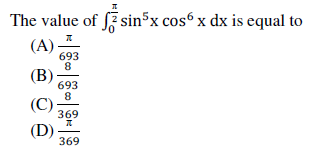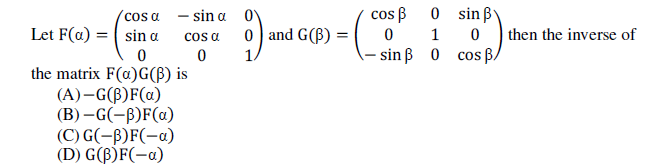Solved Question Paper of Recruitment Test (Computer Based Recruitment Test) conducted by UPSC for the Post of Air Safety Officer in the Office of the Directorate General of Civil Aviation held on 11.08.2019.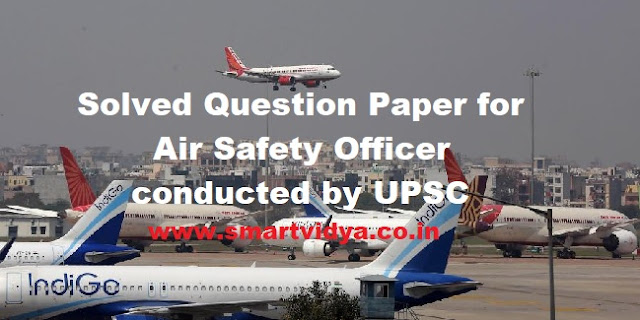1.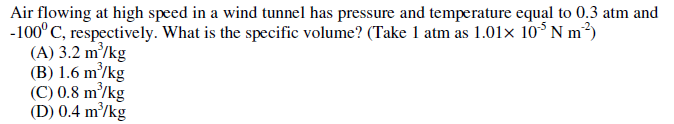2. What is the indication of last two digits in an early NACA 2412 airfoil series?
(A) maximum camber number
(B) maximum thickness
(C) maximum chord
(D) maximum diameter

3. Which type of drag does arise from the resolved components of the tangential stresses on the surface of the body, when viscous fluid flows along the surface?
(A) Trailing vortex drag
(B) Wave drag
(C) Surface friction drag
(D) Boundary layer normal-pressure drag

4. Which one of the following statements is true for the airplane performance conditions that
occur at maximum Lift/Drag ratio?
(A) Maximum range of propeller-driven airplanes
(B) Maximum flow of air
(C) Maximum fuel consumption
(D) Maximum surface area for airplanes

5. An airfoil has the root chord of 1.8 m and tip chord of 0.9 m. What is the taper ratio?
(A) 2
(B) 1
(C) 0.5
(D) 0.25

6.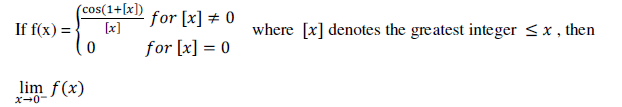(A) is equal to 0
(B) is equal to -1
(C) does not exist
(D) is equal to 2

7. Consider the following statements regarding the forces acting on an airplane in flight:
1. Thrust, drag, lift and weight are the forces on an airplane in flight.
2. The airplane is said to be in state of equilibrium, when thrust and drag are equal
and opposite.
3. If weight is greater than lift, the airplane will sink.
4. If thrust is greater than drag, the airplane will decelerate.

Which of the above statements are correct?
(A) 1, 2 and 3 only
(B) 1, 2 and 4 only
(C) 2, 3, and 4 only
(D) 1, 2, 3 and 4

8. Let f ∶ R → R and g ∶ R → R be defined by g(x) = xf(x) then
(A) g is one-one if f is one-one
(B) g is a differentiable function
(C) g is integrable over any finite interval [a, b]:a, b ∈ R
(D) g is differentiable at 0 if f is continuous at 0